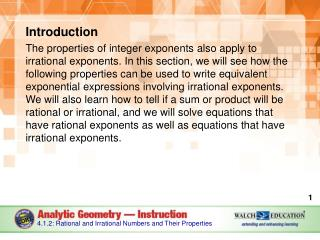DownloadDownload PresentationIntroduction

# Introduction

Télécharger la présentation## Introduction

- - - - - - - - - - - - - - - - - - - - - - - - - - - E N D - - - - - - - - - - - - - - - - - - - - - - - - - - -
##### Presentation Transcript

1. Introduction The properties of integer exponents also apply to irrational exponents. In this section, we will see how the following properties can be used to write equivalent exponential expressions involving irrational exponents. We will also learn how to tell if a sum or product will be rational or irrational, and we will solve equations that have rational exponents as well as equations that have irrational exponents. 4.1.2: Rational and Irrational Numbers and Their Properties

2. Key Concepts An irrational number is a number that cannot be expressed as the ratio of two integers. In other words, it cannot be written as a fraction that has whole numbers for both the numerator and denominator. The decimal representations of irrational numbers neither end nor repeat. The root of a number for which there is no exact root is an irrational number, such as Addition and multiplication are closed operations within integers, meaning that the sum of any integers is an integer, and the product of any integers is also an integer. 4.1.2: Rational and Irrational Numbers and Their Properties

3. Key Concepts, continued Equations involving a power can be solved by taking a root, and equations involving a root can be solved by raising to a power. In order to solve an equation this way, one side of the equation must be a single variable and the other side must be a quantity. If the exponent is rational, raise both sides to the multiplicative inverse (reciprocal) of the fraction. 4.1.2: Rational and Irrational Numbers and Their Properties

4. Key Concepts, continued If the expression is written using a power and a root, it can be rewritten using a rational exponent and then solved using the multiplicative inverse of the rational fraction. Or, each operation, the root and the power, can be “undone” in separate steps. The same operation must be performed on both sides of the equation. 4.1.2: Rational and Irrational Numbers and Their Properties

5. Key Concepts, continued In the following equations, x is the variable and a, b, m, and n represent integers, with m ≠ 0 and n ≠ 0. 4.1.2: Rational and Irrational Numbers and Their Properties

6. Key Concepts, continued 4.1.2: Rational and Irrational Numbers and Their Properties

7. Key Concepts, continued The sum of two rational numbers is a rational number. The product of two rational numbers is a rational number. The sum of a rational number and an irrational number is an irrational number. The product of a rational number and an irrational number is an irrational number. 4.1.2: Rational and Irrational Numbers and Their Properties

8. Common Errors/Misconceptions incorrectly categorizing a root as irrational when an exact root exists incorrectly categorizing a repeating decimal as irrational incorrectly raising a power to a power when solving an exponential equation trying to solve an exponential equation without first isolating the variable confusing the rules for the Power of a Power Property, the Power of a Product Property, and the Product of Powers Property 4.1.2: Rational and Irrational Numbers and Their Properties

9. Guided Practice Example 1 Simplify the expression 4.1.2: Rational and Irrational Numbers and Their Properties

10. Guided Practice: Example 1, continued Identify which property can be used to simplify the expression. This is the product of two exponential expressions with the same base. Use the Product of Powers Property to simplify. 4.1.2: Rational and Irrational Numbers and Their Properties

11. Guided Practice: Example 1, continued Apply the property to simplify the expression. The Product of Powers Property states that if the bases are the same, the expression can be written as the single base raised to the sum of the powers. ✔ 4.1.2: Rational and Irrational Numbers and Their Properties

12. Guided Practice: Example 1, continued 4.1.2: Rational and Irrational Numbers and Their Properties

13. Guided Practice Example 2 Simplify the expression 4.1.2: Rational and Irrational Numbers and Their Properties

14. Guided Practice: Example 2, continued Identify which property can be used to simplify the expression. This is the quotient of two exponential expressions with the same base. Use the Quotient of Powers Property to simplify. 4.1.2: Rational and Irrational Numbers and Their Properties

15. Guided Practice: Example 2, continued Apply the property to simplify the expression. The Quotient of Powers Property states that since the bases are the same, the expression can be written as the base raised to the difference between the power of the numerator and the power of the denominator. If the exponent is negative, the Negative Exponent Property can be used to rewrite the expression. ✔ 4.1.2: Rational and Irrational Numbers and Their Properties

16. Guided Practice: Example 2, continued 4.1.2: Rational and Irrational Numbers and Their Properties